# In the following figure, what is the value of the potential at points a and b?

In the following figure, what is the value of the potential at points a and b?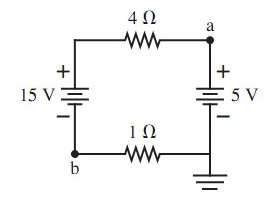Concepts and reason
The concept use to this problem is Kirchhoff’s law and potential between two points.
Initially, apply Kirchhoff’s loop law in the circuit. Then, find the potential between the points a and c. Finally, find the potential at point b.

Fundamental
The expression for the Kirchhoff’s voltage law is as follows: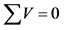Here, V is the potential.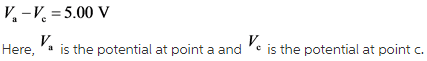The following is the circuit diagram showing the direction of current as follows: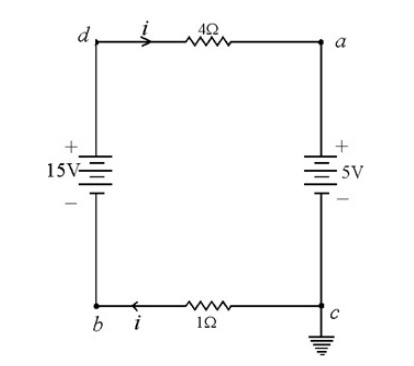Explanation: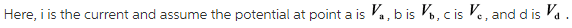The potential at point c, that is, the lower right point is equal to,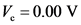Therefore, applying the Kirchhoff’s voltage in the circuit as follows: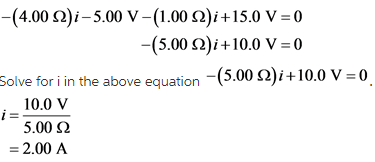Explanation:
According to the Kirchhoff’s voltage law, the sum of the voltages meeting at a single point is equal to zero. And the voltage is equal to the product of the current and the resistance in the circuit.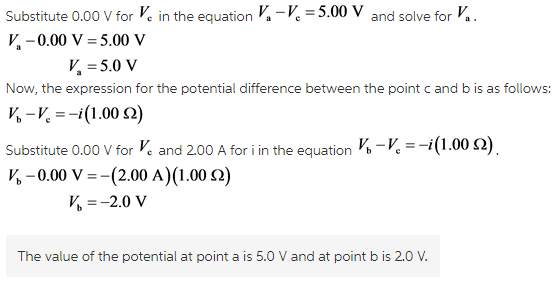Explanation:
The current is flowing through the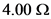resistor at point a from left to right and the current is flowing through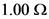resistor at point b from right to left.